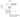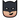# The Pooling UnitPart Zero - The Introduction

Part One - The Architecture Outline

Part Two - The Convolution Engine

Part Three - The Activation Function

Part Four - The Pooling Unit

Part Five - Adding fixed-point number support to our design

Part Six - Generating data and automating the Verification Process

Part Seven - Creating a multi-layer neural network in hardware.

## What is pooling?

The pooler is the part of this design that implements the Max Pooling operation. The animation below shows you what a max-pooling operation is. Basically, it is a particular method of down-sampling data at various stages of the Neural Network in order to reduce the number of parameters involved. this can serve as a good quick introduction to the mathematical process of pooling.One design aspect that we need to keep in mind is that the pooler has also been designed in a pipelined streaming type of architecture in order to complement the Convolver. That is, data coming out of the convolver can be continuously fed into the pooler at every clock cycle and output shall appear after a certain number of clock cycles, this output will be a downsampled version of the input. i.e the output of the convolver.

Understandably, the output of the Max pooler shall be a fraction of the size of its input. This depends on how big the pooling window is. However, most of the commonly used neural networks do not have pooling windows larger than of dimensions 2X2 since anything bigger than that becomes too destructive an operation and results in significant loss of information.

Here is the code for the Max pooler: It is mostly a bunch of control statements that generate control signals for all the individual units in the pooler based on certain conditions that vary with the values of `N`, `K`, and `P`.

## Code

NOTE: Currently this module only implements the max-pooling function since it is the most commonly used one in almost every neural network. However, average pooling is another scheme that is gaining popularity today. That too shall be implemented in the coming time.

``````//file: pooler.v
//this is the top module of the pooling unit and it instantiates several sub-blocks which are explained further in the article
`timescale 1ns / 1ps

module pooler #(
parameter m = 9'h00c, //size of input image/activation map (post convolution)
parameter p = 9'h003, //size of pooling window
parameter N = 16,     //total bitwidth of data
parameter Q = 12,     //number of fractional bits in the Fixed Point representation
parameter ptype = 0,  //ptype = 0 -> max pooling, ptype = 1 -> average pooling
parameter p_sqr_inv = 16'b0000010000000000 //this parameter is needed in average pooling case 												where the sum is divided by p**2.
//It needs to be supplied manually and should be equal 											  to (1/p)^2 in whatever the
//(Q,N) format is being used.
)(
input clk,
input ce,
input master_rst,
input [N-1:0] data_in,
output [N-1:0] data_out,
output valid_op,               //output signal to indicate the valid output
output end_op                  //output signal to indicate when all the valid outputs have been
//produced for that particular input matrix
);

wire [1:0] sel;
wire [N-1:0] comp_op;
wire [N-1:0] sr_op;
wire [N-1:0] max_reg_op;
wire [N-1:0] div_op;
wire ovr;
wire [N-1:0] mux_out;
wire temp2;
reg [N-1:0] temp;

control_logic2 #(m,p) log(          //This block is the brains of this pooling unit. It generates
//the various signals needed to control all the other blocks
.clk(clk),                      //in the pooling unit.
.master_rst(master_rst),
.ce(ce),
.sel(sel),
.rst_m(rst_m),
.valid_op(valid_op),
.global_rst(global_rst),
.end_op(end_op)
);

comparator2 #(.N(N),.ptype(ptype)) cmp(  //ptype = 0 -> This comparator outputs the maximum of
.ip1(data_in),                       //the two inputs.
.ip2(mux_out),                       //ptype = 1 -> This comparator outputs the sum of the
.comp_op(comp_op)                    //two inputs.
);

max_reg #(.N(N)) m1(                     //A simple register to hold the current maximum/sum
.clk(clk),                           //value. It can also be reset to zero
.din(comp_op),
.rst_m(rst_m),
.global_rst(temp2),
.master_rst(master_rst),
.reg_op(reg_op)
);

variable_shift_reg #(.WIDTH(N),.SIZE((m/p))) SR (
.d(comp_op),                                 // input [N-1 : 0] d
.clk(clk),                                   // input clk
.rst(global_rst && master_rst),              // input sclr
.out(Q)                                      // output [N-1 : 0] q
);

input_mux #(.N(N)) mux(                   //the multiplexer that controls one input of the
.ip1(Q),                              //comparator (refer post title image)
.ip2(reg_op),
.sel(sel),
.op(mux_out)
);

qmult #(N,Q) mul (max_reg_op,p_sqr_inv,div_op,ovr);  //fixed point multiplier

assign data_out = ptype ? div_op : max_reg_op; //for average pooling, we output the sum divided by 												 p**2

endmodule``````

Despite looking a bit taunting at first, most of the blocks in the above module are pretty simple and straight forward. Their operations are just as mentioned in the comment next to the module instantiation. I shall be skipping the code for these modules for brevity sakes. You can readily find all the code and test benches at the Github repo of this site.

However, the module that says control_logic2 is the most important sub-module of the pooling unit. It generates all the control signals shown in the title diagram of this page. These signals tell all the other modules in the pooling unit how to behave and what data to send where. It is very important that we dig deep into the functioning of this control logic module.

``````//file: control_logic2.v
`timescale 1ns / 1ps

// The following are the various cases that arise as the pooling window moves over the input matrix
// each case requires a different kind of behaviour from the other modules in the pooler.
//NOTE: here 'max value' => maximum of all the values withing the pooling window

//1. normal case : just store the max value in the register.
//2. end of one neighbourhood: store the max value to the shift register.
//3. end of row: store the max value in the shift register and then load the max register from the shift register.
//4. end of neighbourhood in the last row: make output valid and store the max value in the max register.
//5. end of last neighbourhood of last row: make op valid and store the max value in the max register and then reset the entire module.

//SIGNALS TO BE HANDLED IN EACH CASE
//CASE               1       2       3       4       5
//1. load _sr       low     high    high    high    low
//2. sel            low     low     high    high    low
//3. rst_m          low     high    low     low     low
//4. op_en          low     low     low     high    high
//5. global_rst     low     low     low     low     high

module control_logic(
input clk,                        //clock
input master_rst,                 //reset to initialize every module
input ce,                         //clock-enable
output reg [1:0] sel,             //selection output that connects to the multiplexer input select lines
output reg rst_m,                 //signal to reset the maximum register
output reg op_en,                 //signal to tell the outside world when the output is valid
output reg load_sr,               //signal to enable the clock for the shift register
output reg global_rst,            //signal to reset all the othe modules except the control_logic
output reg end_op                 //signal to indicate end of all outputs for a particular input matrix
);

parameter m = 9'h004;             //size of input matrix is m X m
parameter p = 9'h002;             //size of the pooilng window is p X p
integer row_count =0;             //the entire module works based on the row and column counters
integer col_count =0;             //that tell it where exactly the window is at each clock cycle
integer count =0;                 //the master counter that increments and resets row_count and col_count
integer nbgh_row_count;           //this counter keeps track of the number of neighbourhoods (pooling windows) completed

always@(posedge clk) begin
if(master_rst) begin
sel <=0;
rst_m <=0;
op_en <=0;
global_rst <=0;
end_op <=0;
end
else begin
if(((col_count+1)%p !=0)&&(row_count == p-1)&&(col_count == p*count+ (p-2))&&ce)   //op_en
begin
op_en <=1;
end
else begin
op_en <=0;
end
if(ce)
begin
if(nbgh_row_count == m/p)    //end_op
begin
end_op <=1;
end
else
begin
end_op <=0;
end

if(((col_count+1) % p != 0)&&(col_count == m-2)&&(row_count == p-1)) //global_rst and pause_ip
begin
global_rst <= 1;                           //  (reset everything)
end
else
begin
global_rst <= 0;
end

//end

if((((col_count+1) % p == 0)&&(count != m/p-1)&&(row_count != p-1))||((col_count == m-1)&&(row_count == p-1)))     //rst_m
begin
rst_m <= 1;
end
else
begin
rst_m <= 0;
end

if(((col_count+1) % p != 0)&&(col_count == m-2)&&(row_count == p-1))
begin
sel <= 2'b10;
end
else
begin
if((((col_count) % p == 0)&&(count == m/p-1)&&(row_count != p-1))|| (((col_count) % p == 0)&&(count != m/p-1)&&(row_count == p-1))) begin     //sel
sel<=2'b01;
end
else
begin
sel <= 2'b00;
end
end

if((((col_count+1) % p == 0)&&((count == m/p-1)))||((col_count+1) % p == 0)&&((count != m/p-1))) //load_sr
begin
end
else
begin
end
end
end
end

always@(posedge clk) begin            //counters
if(master_rst) begin
row_count <=0;
col_count <=32'hffffffff;
count <=32'hffffffff;
nbgh_row_count <=0;
end
else
begin
if(ce)
begin
if(global_rst)
begin
row_count <=0;
col_count <=32'h0;
count <=32'h0;
nbgh_row_count <= nbgh_row_count + 1'b1;
end
else
begin
if(((col_count+1) % p == 0)&&(count == m/p-1)&&(row_count != p-1)) //col_count and row_count
begin
col_count <= 0;
row_count <= row_count + 1'b1;
count <=0;
end
else
begin
col_count<=col_count+1'b1;
if(((col_count+1) % p == 0)&&(count != m/p-1))
begin
count <= count+ 1'b1;
end
end
end
end
end
end
endmodule``````

## Simulation

The following code presents a very minimalistic test-bench to check the working of the pooler. This test bench has been far extended to cover all possible inputs and can be found in the Github Repo

The test-bench here applies a 2X2 pooling window on the following 4X4 input matrix:

``````+-----------+
|00|01|02|03|
+-----------+
|04|05|06|07|
+-----------+
|08|09|10|11|
+-----------+
|12|13|14|15|
+-----------+``````

You can easily guess what the output matrix should look like:

``````+-----+
|05|07|
+-----+
|13|15|
+-----+``````

Let's see if our design is working!

``````//file: pooler_tb.v

`timescale 1ns / 1ps

module pooler_tb();

reg clk,ce;
reg [31:0] data_in;
reg master_rst;
wire [31:0] data_out;
wire valid_op;
wire end_op;
parameter clkp = 40;
integer i;

pooler #(9'h4,9'h2) dut(
.clk(clk),
.ce(ce),
.master_rst(master_rst),
.data_in(data_in),
.data_out(data_out),
.valid_op(valid_op),
.end_op(end_op)
);

initial
begin
clk = 0;
ce = 0;
data_in = 0;
master_rst = 0;
#100;
master_rst = 1;
#clkp;
master_rst  = 0;
#10;
ce = 1;
for(i = 0; i<25;i = i+1)
begin
data_in = i;
#clkp;
end
end
always #(clkp/2) clk = ~clk;
endmodule``````

On simulating this test-bench on xilinx vivado, we get the following result:As is visible, the valid_op signal goes high only at the correct outputs. i.e the maximum values in the window

All the design files along with their test benches can be found at the Github Repo

Published under ,  on .

Last updated on .### Batman

I'm Batman, a silent nerd and a watchful engineer obsessed with great Technology. Get in touch via the Discord community for this site

Like what you are reading? Let me send the latest posts right to your inbox!

Free. No spam. Unsubscribe anytime you wish.